International Journal of Mechanical Engineering and Applications
Volume 4, Issue 5, October 2016, Pages: 189-198

Research/Technical Note

Analysis of a Dual-Mode Scramjet Engine Isolator Operating From Mach 3.5 to Mach 6

Vu Ngoc Long1, Luu Hong Quan1, Nguyen Phu Hung2, Le Doan Quang3

1Department of Aeronautical and Space Engineering, School of Transportation Engineering, Hanoi University of Science and Technology, Hanoi, Vietnam

2The Ministry of Science and Technology, Hanoi University of Science and Technology, Hanoi, Vietnam

3Faculty of Aviation Technologies, Vietnam Aviation Academy, Ho Chi Minh City, Vietnam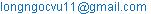(V. N. Long)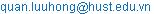(L. H. Quan)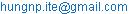(N. P. Hung)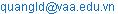(L. D. Quang)

Vu Ngoc Long, Luu Hong Quan, Nguyen Phu Hung, Le Doan Quang. Analysis of a Dual-mode Scramjet Engine Isolator Operating from Mach 3.5 to Mach 6. International Journal of Mechanical Engineering and Applications. Vol. 4, No. 5, 2016, pp. 189-198. doi: 10.11648/j.ijmea.20160405.14

Received: August 28, 2016; Accepted: September 10, 2016; Published: September 29, 2016

Abstract: Isolator is an important component of the hypersonic dual-mode scramjet engine, which plays a critical role on the stability of the engine. Flow structure inside an isolator is quite complicated due to separation zones and shock train. The main function of an isolator is to prevent the separated flow from deviating outside the engine, causing it to stop working. This paper will present a mathematical model of the flow through an isolator, then carry out theoretical flow calculation and CFD simulation in order to determine the length and the operational mode of the isolator of a dual-mode scramjet engine operating from Mach 3.5 to Mach 6. The theoretical and CFD results will also be compared to verify the mathematical model.

Keywords: Dual-mode Scramjet, Isolator, Shock Train, CFD

Contents

1. Introduction

Ramjet engine and scramjet engine respectively are supersonic and hypersonic engines which use shock waves to compress incoming air flow instead of compressor. The core difference between the two is the speed of the flow entering the combustor. Aircrafts with ramjet or scramjet engine cannot take off on their own and need to be propelled to an initial speed in order for the engine to work. To design an aircraft that is capable of hypersonic speed and can take off on its own, a hybrid, multi-stage engine is used. The first stage implements the conventional turbojet engine, which can take the aircraft up to Mach 3.5 . From Mach 3.5 to Mach 5, ramjet cycle is used and for flying speed from Mach 5 and up, scramjet cycle is used. To simplify the structure of the hybrid engine, a configuration that can operate in both ramjet mode and scramjet mode – the dual-mode scramjet engine is needed. The idea of dual-mode scramjet was introduced by Curran and Stull by 1963 [2,3]. This is an engine that combined the two modes in one structure whose shape is the same as a pure scramjet engine. One of the concept aircraft implementing dual-mode scramjet engine is Lockheed Martin SR-72, also known as the Son of Blackbird, which has a design speed of Mach 6 and is envisaged entering service by 2030 .

The combustion process generates high pressure in front of the combustor, i.e. behind the isolator. This high level of pressure creates an adverse pressure gradient. This in turn causes the flow to separate and a system of shock waves to form. This system of shock waves is called the shock train. To prevent the shock train and the separated region from deviating outside of the engine through the inlet, where air is supposed to be coming into the engine, causing the engine to unstart (the state where the engine is not operational due to lack of incoming air flow rate), a component between the inlet and the combustor is needed. Such component is called the isolator.

The configuration of an isolator is fairly simple: a constant area duct. The length of the duct is determined from the length of the shock train, it has to be greater in order to successfully contain the shock train.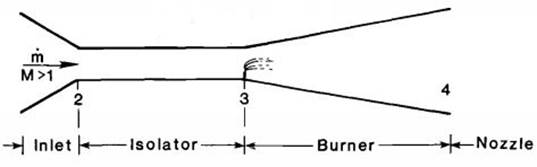Figure. 1. Station designations of the components of a dual-mode scramjet engine.

The flow in the isolator will behave according to flow properties at the front (position 2) and the back (position 3) of the isolator. It has been discovered that the isolator cannot operate with any pair of flow properties at position 2 and 3, there is a limit: With a certain M2, the isolator cannot generate a M3 below the value equal to the downstream Mach number of a normal shock wave with the upstream Mach number M2. In other word, for a predetermined M3, the condition under which the isolator and the whole engine can operate is M2>M2x, with M2x being the Mach number of the flow that will create the Mach number M3 after a normal shock wave.

When the limit mentioned above is satisfied, the isolator can operate in either one of the three following modes [2,3,5]:

Shock free mode: This is the mode where flow properties are unchanged all the way through the isolator and there is no shock train in it. The isolator in this case can be omitted.

Oblique shock train mode: When P3 is greater than P2 but the difference between the two is not too high, an oblique shock train will form in the isolator.

Normal shock train mode: When the difference between P3 and P2 is high enough, a normal shock train will form in the isolator.

Therefore, there are two major problems: determining the suitable length of the isolator and the mode it operates in.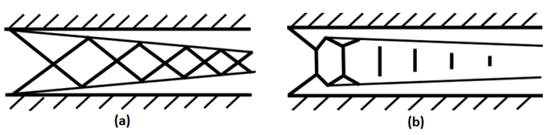Figure. 2. Isolator in: (a) oblique shock train mode and (b) normal shock train mode.

2. Theoretical Calculation

For the preliminary study of flow through the isolator, the following assumptions are made:

Heat transfer between the flow and the isolator wall is neglected. The flow through the isolator is assumed to be adiabatic.

Air is assumed to be perfect gas with constant gas index and specific heat capacity at constant pressure.

Skin friction coefficient is assumed to be constant.

After many experiments with different Mach number, Reynolds number and isolator geometries, Ortwerth  determined that the distribution of pressure along the isolator can be dictated by the following formula: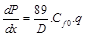(1)

Where:

D is the hydraulic diameter (m).

q is the dynamic pressure of the flow at the position x (Pa).

Cf0 is friction coefficient at the point where flow separation begins. With an ideal duct with zero roughness, this friction coefficient can be calculated using the formula developed by Stuart W. Churchill  as follows: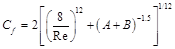(2)

Where: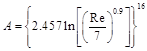,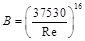The Reynolds number is calculated from flow properties at position 2.

Transform the position variable x to the normalized position variable X: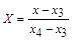and substitute: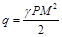into equation (1), the following equation is obtained: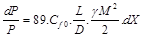(3)

Where L is the shock train length (m).

As the flow is separated, the area of the core flow Ac(X) is not always equal to that of the duct. Therefore, besides M(X), there is a second unknown Ac(X). A system of two differential equations is needed to find flow properties through the isolator.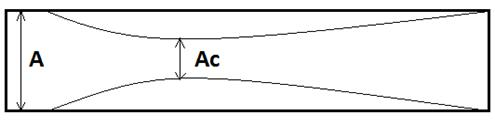Figure. 3. Core flow through the isolator.

Based on the three conservation equations of mass, momentum and energy, Michael K. Smart  has developed the two differential equations as follows: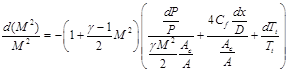(4)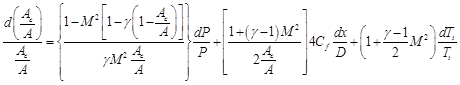(5)

To apply these two equations, we take out the components with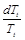to conform with the adiabatic flow assumption.

Substituting equation (3) into equations (4) and (5), two following differential equations are obtained: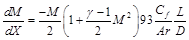(6)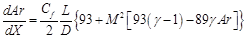(7)

Where Ar is the ratio: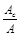.

The above system of two differential equations gives the root M(X), which shows the change of flow Mach number according to the normalized position variable X. P(X) can be calculated from M(X) as follows [2,3]: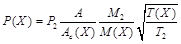(8)

The calculation process for the inlet gives the results as in Table 1. The calculation process for the combustor shows that P3 is equal to 193.217 kPa . From these values, equations (6) and (7) are solved numerically using the 4th order Runge-Kutta method and the results are presented in Table 2:

Table 1. Flow properties after the inlet.

 M0 M2 P2(kPa) Pt2(kPa) Tt2(K) 3.5 2.231 36.515 409.282 747.615 4 2.518 34.472 610.437 915.18 5 3.021 33.018 1286.047 1324.2 6 3.446 33.463 2472.739 1843.36

Table 2. Theoretical result of isolator calculation.

 M0 M3 P3(kPa) Tt3(K) Lshock train (m) 3.5 0.568 193.217 747.615 0.132 4 0.807 193.217 915.18 0.087 5 1.242 193.217 1324.2 0.05 6 1.633 193.217 1843.36 0.033

From Table 2, we can see that the length of the shock train decreases as the Mach number increases. At Mach 3.5, the shock train length is highest, equal to 0.132m. The length of the isolator has to be greater than this value in order for it to be able to contain the shock train. A value of 0.15m is chosen.

3. CFD Simulation

The isolator has a very simple 2D geometry, which is a rectangle whose length is 0.15m and height is 0.025m, corresponding to the throat height of the inlet .

As the model is axis symmetrical, it is cut in half along the axis to minimize the size of the computational fluid domain. Structures mesh is then generated. The element size is smaller from the axis of the isolator to the wall to better capture boundary phenomena. As the mesh is generated in a rectangle geometry, the skewness and orthogonal quality is perfect. Max aspect ratio is quite large (41.152) but still within the limit for a robust solution in Fluent .

The mesh and the boundary conditions are as follows: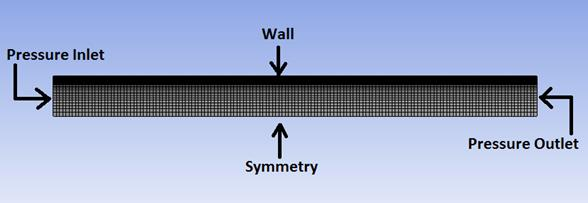Figure. 4. Mesh and boundary conditions.

The values for the boundary conditions are from Tables 1-2.

Two turbulence models are used for a solution steering method. In the first step of the solution, K-Epsilon Realizable is used to determine the y+ of the elements adjacent to the isolator wall. Then, the adapt tool in Fluent is used to chop these elements by the cutcell method so that the y+ is reduced below 1. In the second step, standard K-Omega is used to fully capture the boundary layer phenomenon.

Pressure contours are shown in Figs. 5-8: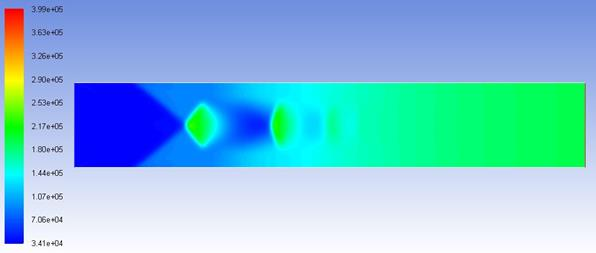Figure 5. Pressure contour at M=3.5.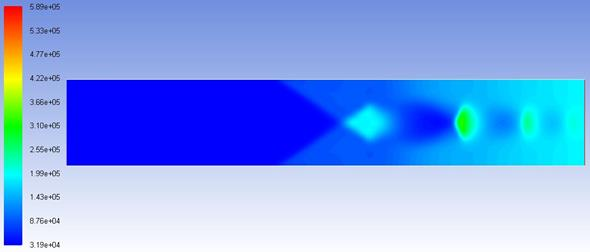Figure 6. Pressure contour at M=4.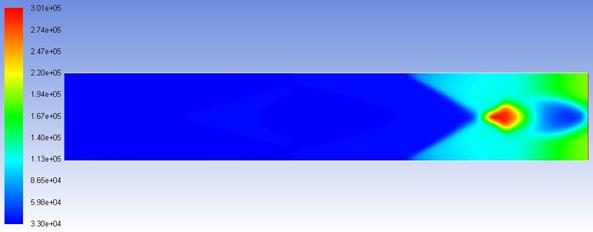Figure 7. Pressure contour at M=5.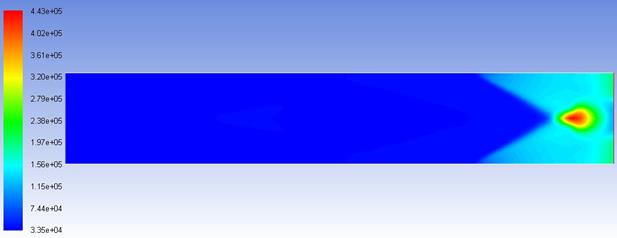Figure 8. Pressure contour at M=6.

Mach number contours are shown in Figs. 9-12: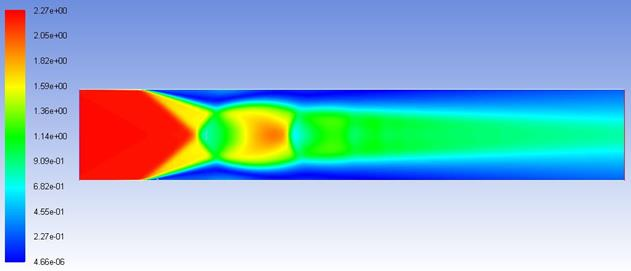Figure 9. Mach number contour at M=3.5.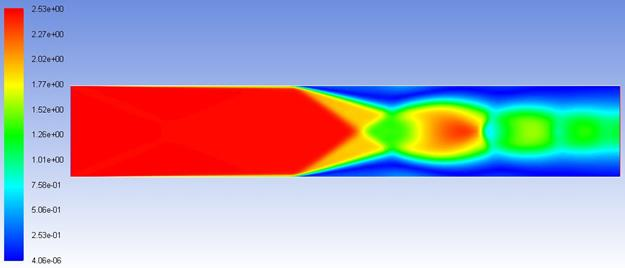Figure 10. Mach number contour at M=4.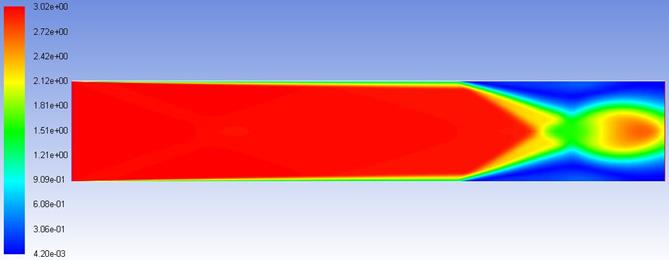Figure 11. Mach number contour at M=5.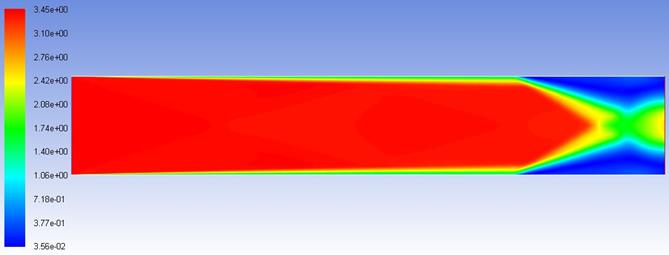Figure 12. Mach number contour at M=6.

Mach number contours are shown in Figs. 13-16: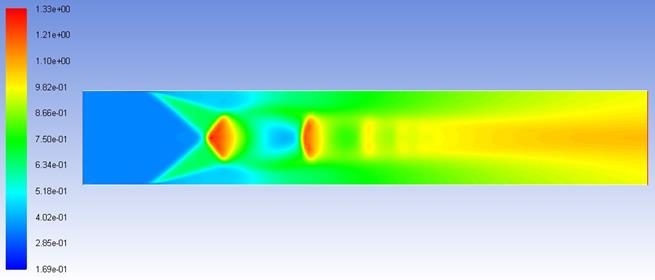Figure 13. Density contour at M=3.5.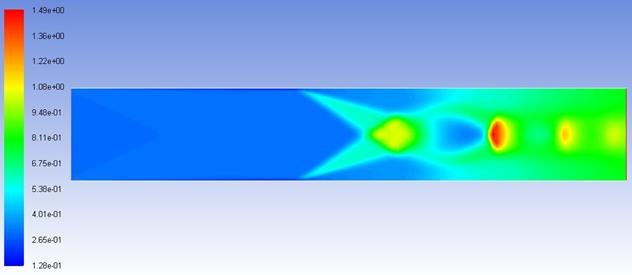Figure 14. Density contour at M=4.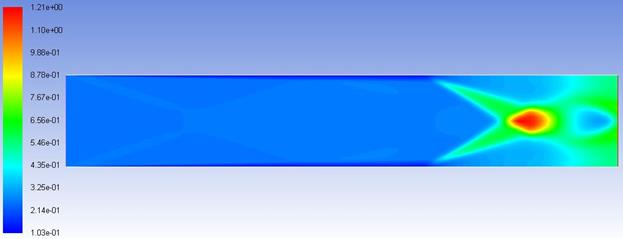Figure 15. Density contour at M=5.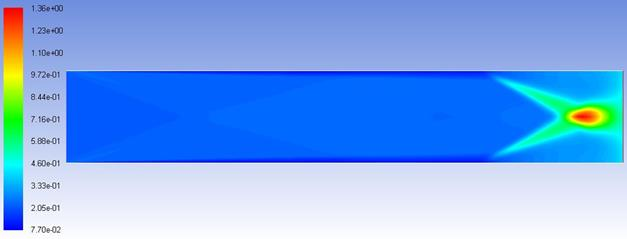Figure 16. Density contour at M=6.

4. Results Comparison

The graphs below show the change in wall pressure of the isolator according to the CFD simulation. The x-coordinate, ranging from 0 to 0.15m, represents the length of the isolator, with position 0 corresponding to its front end. The position where pressure starts to increase is also the starting point of the shock train. From the x-coordinate of that point, we can calculate the length of the shock train.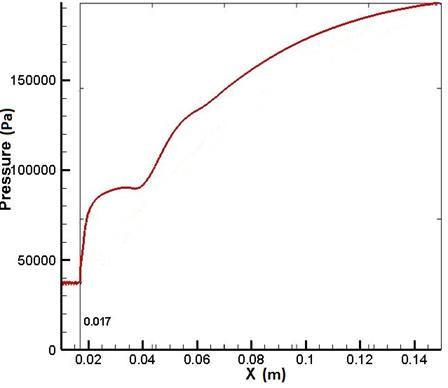Figure 17. Wall pressure of the isolator at M=3.5.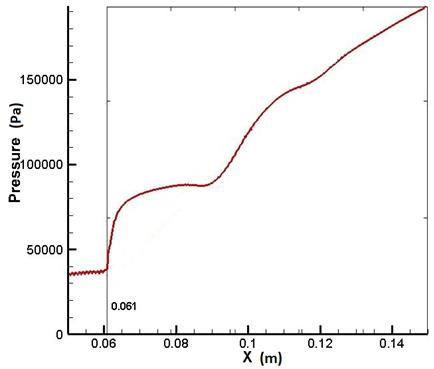Figure 18. Wall pressure of the isolator at M=4.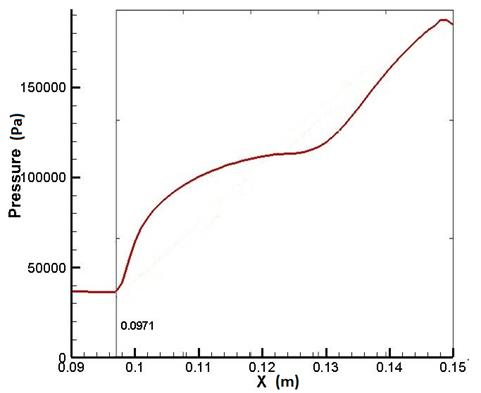Figure 19. Wall pressure of the isolator at M=5.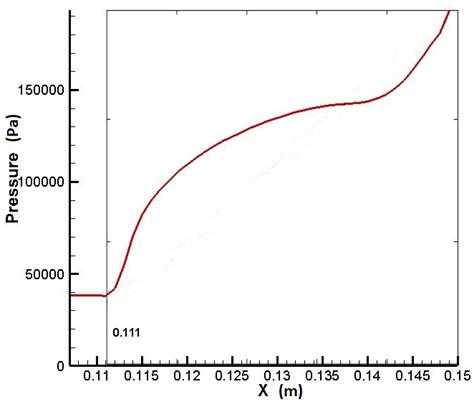Figure 20. Wall pressure of the isolator at M=6.

The shock train length at different free stream Mach numbers according to the theoretical calculation and the CFD simulation along with the errors are as follows:

Table 3. Error of shock train lengths.

 M0 Theoretical results (m) CFD results (m) Error 3.5 0.132 0.133 0.76% 4 0.087 0.089 2.3% 5 0.05 0.0529 5.8% 6 0.033 0.039 18.18%

The main cause of these errors is the assumption that skin friction coefficient is constant throughout the isolator. In fact, it changes depending on the flow properties at each given point.

The error increases at higher free stream Mach number. This is because at Mach 5 and Mach 6, the shock train is short and consists of some of the first shocks. Thus, it is placed in the position near the point where the flow starts to separate. This position has complex shock-boundary layer interaction (this can be seen through the fluctuation of wall pressure: it fluctuates at the beginning of the shock train and becomes stable towards the end), leading to higher value of error.

The CFD contours show initial oblique shocks and normal shocks within the shock train. This can be clearly seen when comparing the shock structure in the Mach number contour at Mach 3.5 with Fig. 2 (b).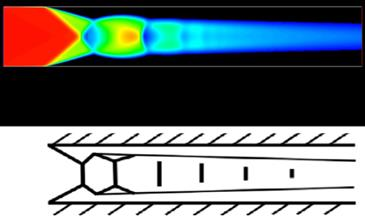Figure 21. Comparison of CFD result and shock structure illustration.

This complex shock structure is originally caused by strong adverse pressure gradient that the flow in the isolator experiences. This makes the region of the flow near the isolator wall separate. The separation region acts as two wedges where two oblique shocks form as the supersonic flow passes through. These two shocks are followed by a train of normal shocks in the core flow. The separated region and the core flow are split by a middle region called the mixing region.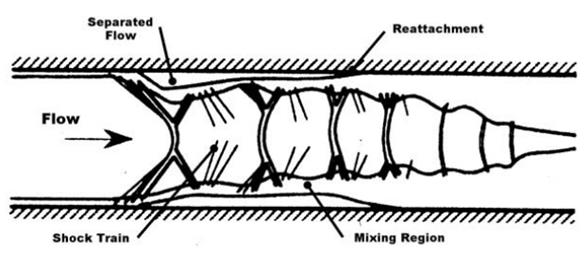Figure 22. Flow structure in an isolator.

5. Conclusion

The pressure and Mach number contours from the CFD simulation show normal shock interfaces at Mach 3.5, meaning that the isolator operates at normal shock train mode. Then, the shock train becomes shorter as the free stream Mach number increases until there are only two oblique shocks in the isolator at Mach 6. This is similar to the theoretical result: the length of the shock train is gradually shorter from Mach 3.5 to Mach 6. The shock train length, which is the key parameter for determining the isolator length, have the error values within an acceptable limit. Therefore, the presented mathematical model is suitable for preliminary estimation of isolator length.

References

1. Marta Marimon Mateu, Study of An Air-breathing Engine for Hypersonic Flight, Technical Report, Universitat Politecnica de Catalunya, Spain, September 2013.
2. Heiser, W.H. and Pratt, D.T., Hypersonic Airbreathing Propulsion, AIAA Education Series, 1994.
3. E.T. Curran, S.N.B. Murthy, Scramjet Propulsion, Vol 189, Progress in Astronautics and Aeronautics, AIAA, 2000.
4. Guy Norris, Skunk Works Reveals SR-71 Successor Plan, Aviation Week & Space Technology, 2013.
5. Christian Max Fischer, Investigation of the Isolator Flow of Scramjet Engines, Ph.D. Thesis, RWTH Aachen Universiry, Germany, 2014.
6. Ortwerth, P.J., Scramjet Vehicle Integration, Scramjet Propulsion, Progress in Astronautics and Aeronautics, AIAA Washington DC, 2001.
7. The Engineering Department, Crane Co., Flow of Fluids through Valves, Fittings and Pipe, Technical Paper No. 410, 2010.
8. Michael K. Smart, Scramjet Isolators, Centre for Hypersonics, The University of Queensland Brisbane 4072, Australia, September 2010.
9. Vũ Ngọc Long, Design of Hypersonic Scramjet Engine Inlet, Graduation Thesis, Hanoi University of Science and Technology, Vietnam, 2016.
10. ANSYS Inc, Introduction to ANSYS Meshing, Release 14.5, 2012.

BiographyNguyen Phu Hung (1976, Vietnam), Associate Professor. PhD in Aeronautic Thermal-Aerodynamic – École Nationale Supérieure de Mécanique et d’Aérotechnique (ENSMA), France. Researcher at the Ministry of Science and Technology, Vietnam and Invited Lecturer at Department of Aeronautical and Space Engineering, School of Transportation Engineering, Hanoi University of Science and Technology, Vietnam. Research Experience: Aerospace propulsion, Aero-thermal Characteristics of Supersonic Flows, Numerical Method in Fluid Mechanics, Design and Fabrication of UAV. About 4 publications in scientific journals and about 16 papers on conference proceedings.Le Doan Quang (1976, Vietnam), Master of Engineering (Aerospace) – Ho Chi Minh University of Technology (HCMUT), Vietnam. PhD student at Department of Aeronautical and Space Engineering, School of Transportation Engineering, Hanoi University of Science and Technology, Vietnam. Acting Dean, Faculty of Aviation Technologies, Vietnam Aviation Academy, Vietnam. Research Experience: Impact of thin wall structure, Scarmjet Engine. About 4 papers on conference proceedings.Luu Hong Quan (1986, Vietnam), PhD in Energy, Thermal and Combustion – École Nationale Supérieure de Mécanique et d’Aérotechnique (ENSMA), France. Lecturer and Researcher at Department of Aeronautical and Space Engineering, School of Transportation Engineering, Hanoi University of Science and Technology, Vietnam. Research Experience: Fluid Mechanics, Aerodynamics, Thermal – Combustion, Computational Fluid Dynamics, Fluid Structure Interaction. About 6 papers on conference proceedings.Vu Ngoc Long (1993, Vietnam), B.S in Aeronautical and Space Engineering – Hanoi University of Science and Technology (Graduation: June 2016, Senior Project: Hypersonic Air-breathing Engine: Scramjet). Research and Work Experience: Aerodynamics, Hypersonic Propulsion. 1 publication in scientific journal and 1 paper on conference proceeding.
 Contents 1. 2. 3. 4. 5.
Article ToolsAbstractPDF(823K)